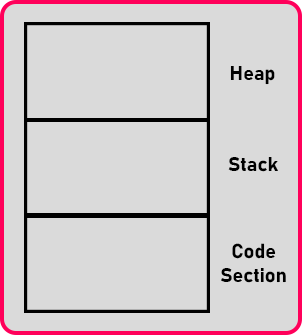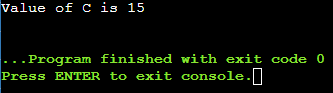# Dynamic Declaration in C++

## Dynamic Declaration in C++ with Examples:

In this article, I am going to discuss Dynamic Declaration in C++ with Examples. Please read our previous article where we discussed Short Circuit in C++ with Examples.

##### Dynamic Declaration in C++:

C++ allows a dynamic declaration of the variables. Let us understand what are the benefits of allowing dynamic declaration.We know memory is divided into three sections and used by the program. The ‘Code Section’ is the place where the program itself resides. Inside ‘Stack’, the memory for the variables will be created during the execution of a program. Let us have the main function and we are declaring some variables inside the main function as follows.
Int main(){
Int a, b, c;
}

Here main will be created inside the ‘Code Section’ and variables will be created inside ‘Stack’. If we need some temporary variable inside any conditional block-like,
If (a > b){
int n;
}
Here we defined that temporary variable inside the ‘if’ block. So, when control enters in this ‘if’ block then a variable of ‘n’ will be created inside ‘Stack’ and when controls exit the ‘if’ block, then the memory of the variable ‘n’ will be free or deleted from the ‘Stack’. So, once the block ends this memory of the temporary variable will go.

Inside the same conditional statement again we need one variable that is ‘x’ that is temporarily inside the if block, then the same memory where ‘n’ was created will be located and utilized for the variable ‘x’ and that X will be there in the memory as long as the ‘if’ block is executing.

So, this one side memory can be used again and again by different variables whereas in C language all the variables must be declared only in the beginning, and whether you are using them towards the program or not it doesn’t matter.

So, some of that may not be useful for the program but they will occupy the memory space so that was the drawback of C programming but in C++ you can declare whenever you require.

int main(){
int a;
int k = (_some_expression_);
if (k < a){
}
}
Now let us have a variable ‘k’ and assign some expression to ‘k’. Now this ‘k’ is used to assign the result of some expression and is only useful in the ‘if’ condition. So, for storing the result we have to declare it outside the ‘if’ block.

But we want the scope of this ‘k’ variable till ‘if’ block is executing after that ‘k’ will be useless. Once we declare it outside the ‘if’ block, it will be there for the whole main function. We want the scope of the ‘k’ variable limited to the ‘if’ block. And we want this to be removed from the memory once this ‘if’ block ends. So how we can do this by writing,
if (int k = _expression_; k < a){
}

Here is the variable inside the block. This is the new future allowed in C++. This is allowed even inside the conditional state when you can declare half declaration and followed by that you can have the condition so the life of this variable will be limited to this ‘if’ block.

Once this block ends, this will be removed from the memory. Now reusing a deleted memory of a variable is very common in C++ programs as that is required for loops. We will study loops in upcoming articles. So, we can efficiently utilize the memory in C++ with the help of dynamic declarations.

##### Program for Dynamic Declaration in C++:
```#include <iostream>
using namespace std;

int main()
{
int a = 10, b = 5;

if (int c = a + b; c > 10)
{
cout << "Value of C is " << c << endl;
}
// declaration inside if conditional statement is the feature of C++17.
// c can't be use outside the if block
return 0;
}
```
###### Output:In the next article, I am going to discuss Switch Case Statements in C++ with Examples. Here, in this article, I try to explain Dynamic Declaration in C++ with Examples and I hope you enjoy this Dynamic Declaration in C++ with Examples article.